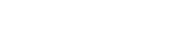# Learn and Understand JavaScript Variables

Hack Reactor

Learn and understand JavaScript variables with this helpful tutorial below. You can also read more about why we're a JavaScript focused bootcamp in this post.

### What Are JavaScript Variables?

Transcription: A variable is simply a place to hold a value. When we use JavaScript variables we often first declare them. When we declare variables we simply say, "Ok, we're going to use a variable and give it a specific name." We do that with the Var keyword.

Let's say we declared a variable called userName. We also could declare a variable called userBattingAverage (for baseball fans).

When we declare the variables we also have to initialize the variables. I'm putting in comments here using //. Comments are ignored by the browser. They're for you, the developer, to make your code more clear.

We initialize the variable by giving them an initial value. Let's give our userBattingAverage an initial value of .312. What we've done down here is called assignment. You commonly call this guy the equal sign, but in this context it's being used as the assignment operator. We're assigning the value Mark Lasoff to the user name variable. We also have the user batting average being assigned the value .312.

Whenever we have an assignment operator, whatever is going on over here is always processed first. For example, if we had "age in dog years," and a dog year is roughly equivalent to seven human years. So let's say we said "age in dog years equals 11x7."

What's exactly going to be assigned to the age in dog years is the value 77. We evaluate the right side first and then we assign it to the variable name.

We also can have what's called a combined declaration and initialization. That would look something like this: Var computerType = macintosh. Here we've declared the variable and we've initialized it. Both of these are equally valid. Different programmers use different styles. It's up to you.

Now that we've assigned these different values we're going to go ahead and put document.write(userName). What this is going to do - notice I didn't put that in quotes, so it's not going to output it literally as a string literal.  It's going to look up the value of userName which is my name, and it's going to output it.

Let's save and open up the file in the browser...and it outputs Mark Lassoff.

We could also say document.write(userBattingAverage). There is .312, notice it appends the leading zero. We can output any variables here. Document.write("age in dog years" + ageInDogYears").

Now you're saying, "wait a minute, you used the quotes there." I'm using here what is commonly called the plus sign but in this context is the concatenation symbol - we're joining this text with the variable, which is going to be ageInDogYears.

### Redeclaring a Variable in JavaScript

What I want you to know before I start doing the document.write is this - we can redeclare a variable in JavaScript. This is not common in other languages. Like this:

Watch what happens. You're probably thinking, "we declared the variable again." But actually redeclaring the variable in JavaScript does not reinitialize the variable. In JavaScript this Var sometimes is not used. I think it's better programming form to use it, but it really doesn't have the same effect that it does in other programming languages.

We've got our assignment operator (=) and our concatenation operator (+)...we can use  a number of different mathematical operators with our variables.

### Calculating Variables in JavaScript

For example, let's say we wanted to do something different here. I'm going to resave this as Var2. I'm going to take out all of this here and declare a couple of variables.

Var atBAts;

Var walks;

Var hits;

atBats = 213;

walks = 19;

hits=59;

I'm going to declare a batting average variable and do some math to figure out what the batting average is. The math is going to be the hits divided by at bats minus walks.

var battingAverage = 59 / (213-19);

Because I put this in parentheses, it will subtract 19 from 213 first. That's precedence. Then we'll divide the result here. Now we can say:

document.write("Batting Average is" + battingAverage);

It will do all the math for us here. Batting average is .304. Pretty good batting average. There are some math functions we can use later to round this off to three places.

### Adding Strings and Numbers in JavaScript

We've looked at mathematical and assignment operators. We also looked at strings initially, but I want to take a look at what happens if we add strings and numbers together, because JavaScript handles strings and numbers a little bit differently.

Let's declare Var playerName = Mark. What would happen if we said:

document.write(playerName + atBats);

It treats the entire thing as a string. atBats is being converted to a string, and then being put together as one long string when we output it.

### JavaScript Variables: Conclusion

So that's variables in JavaScript. If you're coming from another language, variables in JavaScript are not strongly typed. JavaScript automatically differentiates between a string and a number value. We don't have floats and integers and things like that as you might have in other languages.

If this is your first time working with variables I hope this has been easy for you, because variables are important in every programming langauge.